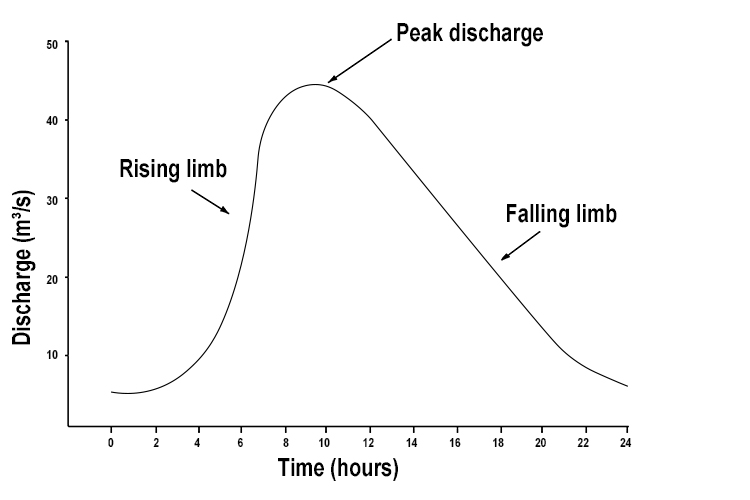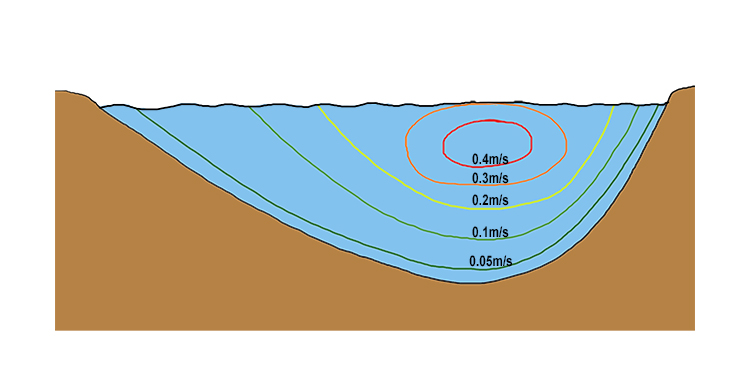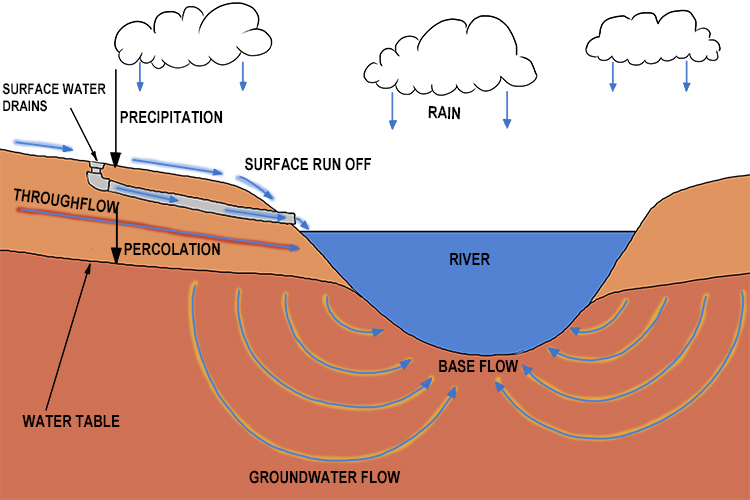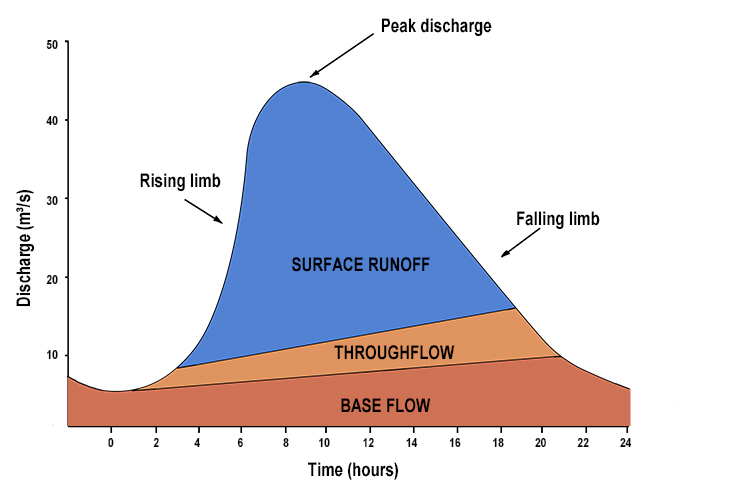# Discharge – The quantity of water that flows past a given point on a stream or river-bank within a given period of timeHe discharged (discharge) his gun as he stood at the start point and thousands (quantity) passed within minutes (time).

This helps to remind you that discharge is the quantity that passes a certain point in a certain time period.

The quantity of water that passes a point in a river is measured in cubic metres per second.The above is a plot of the average number of cubic metres of water passing a point in a river per second, on a day when there was a rainstorm.

The rising limb shows the discharge increasing due to the storm, then peaking, and then falling, reflecting the storm subsiding and ending.

NOTE: We use the word average because, if you look at the cross-section of a river, the velocity of water passing this one point in the river varies:

Velocity of water per second through

a cut section of a riverSome clever calculations are made to work out how this translates to the average velocity or speed of the river per second.

The cubic metres of water per second is calculated by multiplying the cross-sectional area of the river by the average of the velocities shown above.

Cubic metre of water per second = Average velocity (m/s) x Area (m²)

The discharge of water at this point in the river is made up of several elements:This can be plotted on a graph as follows: